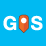# add points in the surface profile with lables with arcpy and matplotlib

877
4
06-05-2018 12:55 AMby
New Contributor

Hi

I have created surface profiles at various locations with the help of the python script from Xander Bakker.

The script works perfect. Now i want to add/show the reference points in each profile (see attachment) . How can i do it? any sggestions?

Tags (3)
4 RepliesbyMVP Legendary Contributor

Is this Xander Bakker‌ 's code?

If so a link, or proper formatting would be nice

What were the results of the test?

What have you tried?by
New Contributor

Hi Dan,

Yes its Xander Bakker's script

The link to the script is: https://community.esri.com/docs/DOC-1973

I am a beginner to python/arcpy. I tried but i think i am missing things like ,extract values to points and position it along the profileline and plot the z values on the profile with looping.

i have ammended the script at:

line 11, 53, 75, and 86

and i got this error

zp.append() # want to append raster values with loop
TypeError: append() takes exactly one argument (0 given)

ammended script is :

``````import arcpy
import os
import matplotlib.pyplot as plt
import numpy
import math

# settings for datasources
linesPath = "lines"
rasterPath = "raster"
pointPath =  "points" # point shapefile

# settings for output profiles PNG's
profileFolder = r"\test_arcpy\profile"
profilePrefix = "ArcPy_"
profilePostfix = "_new.png"
fldName = "hm"

# standard NoData mapping
NoDataValue = -9999

# describe raster
inDesc = arcpy.Describe(rasterPath)
rasMeanCellHeight = inDesc.MeanCellHeight
rasMeanCellWidth = inDesc.MeanCellWidth

# search cursor
fldShape = "SHAPE@"
flds = [fldShape, fldName]
with arcpy.da.SearchCursor(linesPath, flds) as curs:
for row in curs:
feat = row
profileName = row

# read extent of feature and create point for lower left corner
extent = feat.extent
print "Processing: {0}".format(profileName)
pntLL = arcpy.Point(extent.XMin, extent.YMin)

# determine number of rows and cols to extract from raster for this feature
width = extent.width
height = extent.height
cols = int(width / rasMeanCellWidth)
rows = int(height / rasMeanCellHeight)

# create Numpy array for extent of feature
arrNP = arcpy.RasterToNumPyArray(rasterPath, pntLL, cols, rows, NoDataValue)

# create empty arrays for distance (d) and altitude (z) and NAP line (zv)
d = []
z = []
zv = []
zp = [] # voor point shape

# loop through polyline and extract a point each meter
for dist in range(0, int(feat.getLength("PLANAR"))):
XYpointGeom = feat.positionAlongLine(dist, False)
XYpoint = XYpointGeom.firstPoint

# translate     XYpoint to     row, col (needs additional checking)
c = int((XYpoint.X - pntLL.X) / rasMeanCellWidth)
r2 = int((XYpoint.Y - pntLL.Y) / rasMeanCellHeight)
r = rows - r2
if c >= cols:
c = cols-1
if r >= rows:
r = rows-1

# extract value from raster and handle NoData
zVal = arrNP[r,c]
if not zVal == NoDataValue:
d.append(dist)
z.append(arrNP[r,c])
zv.append(0)
zp.append() # want to append raster values with loop

# define output profile filename and figure size settings
elevPNG = profileFolder + os.sep + profilePrefix + profileName + profilePostfix
fig = plt.figure(figsize=(10, 3.5)) # inches

# plot profile, define styles
plt.plot(d,z,'r',linewidth=0.75)
plt.plot(d,z,'ro',alpha=0.3, markersize=3)
plt.plot(d,zv,'k--',linewidth=0.5)
plt.plot(d,zp,'g',alpha=0.3, markersize=3)
plt.xlabel('Distance from start')
plt.ylabel('Elevation')
plt.title('Profile {0} using Python matplotlib'.format(profileName))

# change font size
plt.rcParams.update({'font.size': 8})

# save graph as PNG
fig.savefig(elevPNG, dpi=300)

# delete some objects
del arrNP, XYpointGeom, XYpoint
print "PNGs stored in: {0}".format(profileFolder)

‍‍‍‍‍‍‍‍‍‍‍‍‍‍‍‍‍‍‍‍‍‍‍‍‍‍‍‍‍‍‍‍‍‍‍‍‍‍‍‍‍‍‍‍‍‍‍‍‍‍‍‍‍‍‍‍‍‍‍‍‍‍‍‍‍‍‍‍‍‍‍‍‍‍‍‍‍‍‍‍‍‍‍‍‍‍‍‍‍‍‍‍‍‍‍‍‍‍‍‍‍‍‍‍‍‍‍‍‍‍‍‍‍‍‍‍‍‍‍‍‍‍‍‍‍‍‍‍‍‍‍‍‍‍‍‍‍‍‍‍‍‍‍‍‍‍‍‍‍‍‍‍‍‍‍‍‍‍‍‍‍‍‍‍‍‍‍‍‍‍‍‍‍‍‍‍‍‍‍‍‍‍‍‍‍‍‍‍‍‍‍‍‍‍‍‍‍‍‍‍‍‍‍``````byEsri Esteemed Contributor

Glad to hear the the script is working (at least for part of what you want to achieve). I assume that the zp list will need to be filled with data to be able to add it to the plot.  Since zp will have only a few values, you will need to determine when the values have to be added.

Are the reference values located exactly on the lines? What is the source for these points? To plot the points to location (xy) need to be translated to a distance from start.by
New Contributor

Hi Xander,